Categories

## 2 + 2 with neural networks

Some weeks ago, I was at a get together with my old university friends that we call “the hack day”. It usually revolves around drinking lots of coffee and soft drinks, eating loads of chips and candy, as well as working on the occasional masterpiece project like Zombie Hugs.

At the end of the day, one of us (I cannot remember who now) mentioned how useful it would be to have a neural network that could add numbers together.

The remark was meant as a joke, but it got me thinking, and on the way home on the train, I pieced together some code for creating a neural network that could perform addition on two numbers between 0 and 9. Here’s the original code.

Warning: The rest of this post is probably going to be a complete waste of your time. The whole premise for this post is based on a terrible idea and provides no value to humanity. Read on at your own risk :-)

### Making addition more interesting

It is worth mentioning that it is actually trivial to make a neural network add numbers. For example, if we want to add two numbers, we can construct a network with two inputs and one output, set the weights between input and output to 1, and use a linear activation function on the output, as illustrated below for 20 + 22: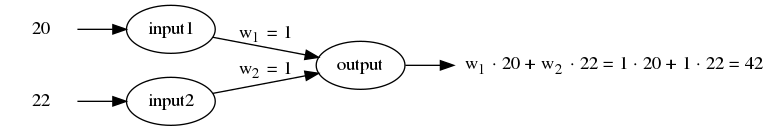Illustration of neural network for adding two numbers, with an example for 20 + 22.

It is not really the network itself that performs addition. Rather, it just takes advantage of the fact that a neural network uses addition as a basic building block in its design.

Things get more interesting if we add a hidden layer and use a non-linear activation function like a sigmoid, thereby forcing the output of the hidden layers to be a list of numbers between 0 and 1. The final output is still a single number which is a linear combination of the output of the hidden layer. Here is a network with 4 hidden nodes as an example: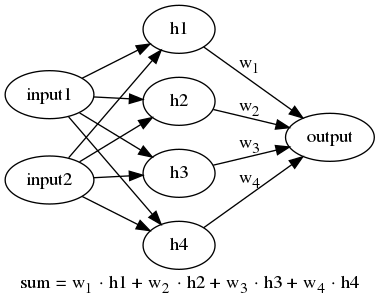Example of neural network with one hidden layer with four nodes (h1-h4).

When we ask a computer to perform 2 + 1, the computer is really doing 10 + 01 (2 is 10 in binary and 1 is 01). I had this thought at the back of my mind, that the neural network might “discover” an encoding in the hidden layer which was close to the binary representation of the input numbers.

For example, for the 4-node hidden layer network illustrated above, we could imagine the number from input1 being encoded in h1 and h2 and the number for input2 being encoded in h3 and h4.

For 2 + 1, the four hidden nodes would then be 1, 0, 0 and 1, and the final output would convert binary to decimal (2 and 1) and add the numbers together to get 3 as result: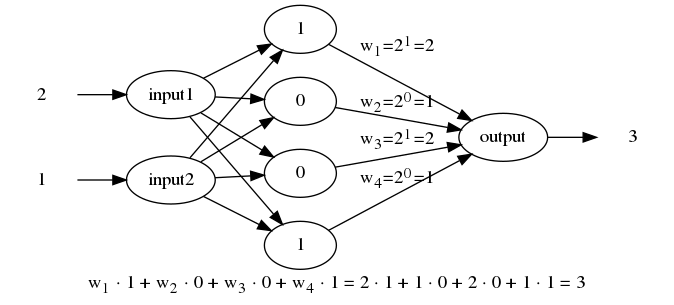Example calculation of 2 + 1 with assumed binary representation in hidden layer.

Since the hidden nodes are restricted to be between 0 and 1, it seemed intuitive to me that a binary representation of the input would be a very effective way of encoding the data, and the network would thus discover this effective encoding, given enough data.

### False assumptions

To be honest, I did not think this through very thoroughly. I should have realized that:

1. The sigmoid function can produce any decimal number between 0 and 1, thus allowing for a very wide range of values. Many of these would probably work well for addition, so it is unlikely it would “choose” to produce zeros and ones only.
2. It is unclear how a linear combination of input weights would produce the binary representation in the first place.

That second point is important. For the 2-bit (2 nodes) encoding, we would have to satisfy these equations (where S(x) is the sigmoid function and w1 and w2 are the weights from the input node to the 2-node hidden layer):

Which weights w1 and w2 would satisfy these equations? Without providing proof, I actually think this is impossible. For example, both S(w2 · 1) ≈ 1 and S(w2 · 2) ≈ 0 cannot be satisfied at the same time. Disregarding the sigmoid function, this is like saying 2x = 0 and x = 1 which is not possible.

### The Experiment

Regardless of the bad idea, false assumption or whatever, I still went ahead and made the following experiment:

• Use two input numbers.
• Use 1, 2, 4, 8 or 16 nodes in the hidden layer.
• Use mean squared error (MSE) on the predicted sum as loss function.
• Generate 10,000 pairs of numbers and their sum for training data.
• Use 20% of samples as validation data.
• Allow the sum of the two numbers to be at most 4, 8 or 16 bits large (i.e. 16, 256 and 65536).
• Train for at most 1000 epochs.

When measuring accuracy, the predicted number is rounded to the nearest integer and is either correct or not. For example, if the network says 2 + 2 = 4.4, it is considered correct, but if it says 2 + 2 = 4.6, it is considered incorrect. 20% accuracy thus means that it correctly adds the two numbers 20% of the time on a test dataset.

Here is a summary of the accuracy and error of these models:

There are a few things that are interesting here:

1. The 1-node network cannot add numbers at all.
2. Networks with 2 or more hidden nodes get high accuracy when adding numbers with a sum of at most 16.
3. All networks perform poorly when adding numbers with a sum of at most 256.
4. All networks have abysmal performance for numbers with a sum of at most 65536.
5. Adding more hidden nodes improves performance most of the time.

Here is a plot of the validation loss for the different networks after each epoch. Training can stop early if the performance does not improve, which explains why some lines are shorter than others: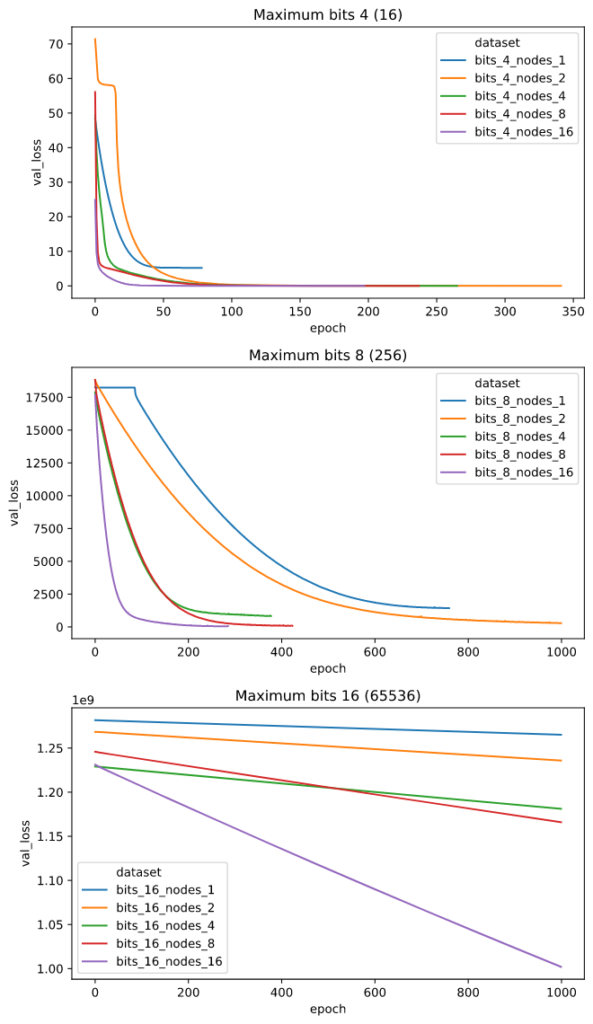Validation loss during training of networks for adding two numbers.

### Exploring prediction errors

Let us look at the prediction error for each pair of numbers. For example, the 1-node network trained on sums up to 16 has an overall accuracy of 20%. When we add 2 + 2 with this network we get 6.42 so the error is 2.42 in this case. If we try a lot of combinations of numbers, we can plot a nice 3D error surface like this:The prediction error for a 1-node hidden layer model trained on sums up to 16.

It looks like the network is good at predicting numbers where the sum is 8 (the valley in the chart), but not very good at predicting anything else. The network is probably overfitting to numbers that sum to 8, because the training data has an overweight of samples that sum to 8.

Adding an extra node brings the accuracy up above 90%. The error surface plot for this network also looks better, but both networks struggle with larger numbers: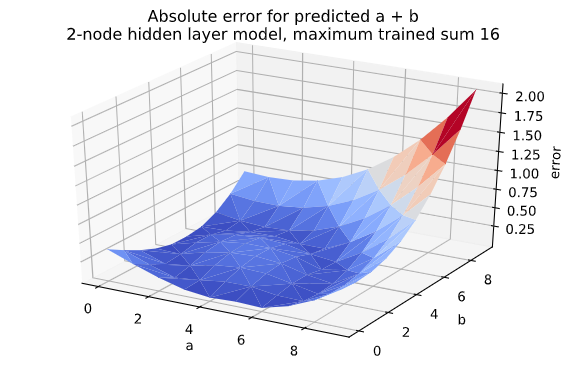The prediction error for a 2-node hidden layer model trained on sums up to 16.

When predicting sums up to 256, the 1-node hidden layer model shows the same error pattern, i.e. a valley (low error) for sums close to 130. In fact, the network only ever predicts values between 78 and 152 (this cannot be seen from the graph), so it really is a terrible model: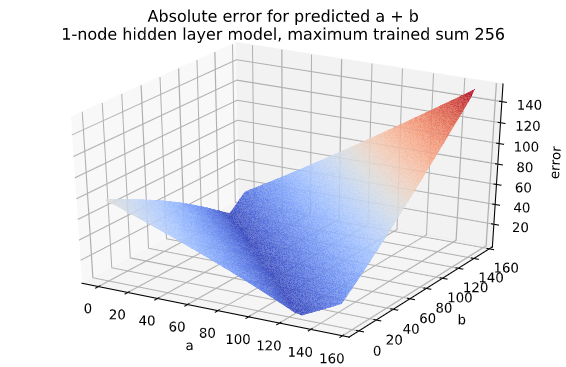The prediction error for a 1-node hidden layer model trained on sums up to 256.

The 2-node hidden layer network does not do much better for sums up to 256 which is expected since the accuracy is just 2%. But it looks fun:The prediction error for a 2-node hidden layer model trained on sums up to 256.

As can be seen in the table above, even the 16-node hidden layer network only had 6% accuracy for sums up to 256. The error plot for this network looks like this:The prediction error for a 16-node hidden layer model trained on sums up to 256.

I find this circular shape to be quite interesting. It almost looks like a moat around a hill. The network correctly predicts some sums between 51 and 180, but there is an error bump in the middle.

For example, for the sum 120, 60 + 60 is predicted as 128.8 (error = 8.8), but 103 + 17 is predicted as 119.9 (error = 0.1) which is correct when rounded. The error curve for numbers that sum to 120 is essentially a cross section of the 3D plot where the hill is more visible: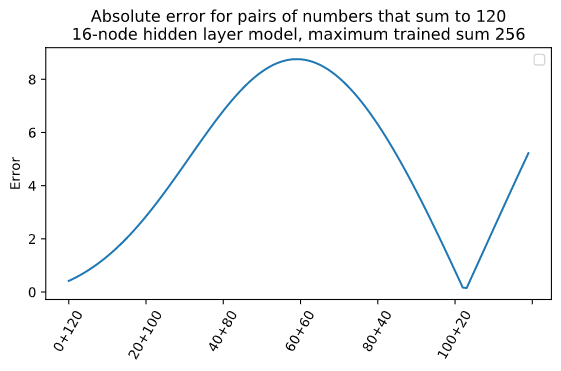Prediction error for numbers that sum to 16-node hidden layer model trained on sums of to 256

I have no idea why this specific pattern emerges, but I find it interesting that it looks similar to the 2-node network when predicting sums up to 16 (the hill and the moat). A more mathematically inclined person could probably provide me with some answers.

Finally, for the networks that were trained on sums up to 65536, we saw abysmal performance in all cases. Here is the error surface for the 16-node network which was the “best” performing one:The prediction error for a 16-node hidden layer model trained on sums up to 65536.

The lowest error this network gets on a test set is the sum 3370 + 329 = 3699 which the network predicts as 3745.5 (error = 46.5).

In fact, the network mostly just predicts the value 3746. As a and b get larger, the hidden layer always produces all 1’s and 0’s (or values very close to 0 and 1), so the final output is always the same. This already starts happening when a and b are larger than around 10 which probably indicates that the network needs more time to train.

### The inner workings of the network

My initial interest was in how the networks decided to represent numbers in the hidden layer of the network.

To keep things simple, let us just look at the 2-node hidden layer network on sums up to 16 since this network produced mostly correct sum predictions.

What actually happens when we predict 2 + 2 with this network is illustrated below. The number above an edge in the graph is the weight between the nodes. There is a total of 6 weights for this network (4 from input layer to hidden layer and 2 from hidden layer to output layer):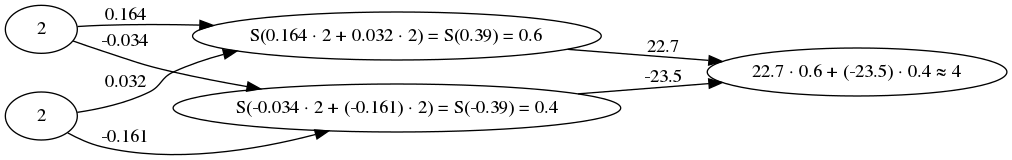The calculation of 2 + 2 for the 2-node hidden layer network trained with sums up to 16.

One thing that might be of interest are the final weights 22.7 and -23.5. The way the network sums numbers is to treat the first hidden node as contributing positively to the sum and the second hidden node to contribute negatively. And they are almost the same.

It turns out that the 4-node hidden layer network works the same way. Here, there are 4 weights between hidden layer and output layer, and these are (rounded) 8, 8, 9 and -25. So we still have the large negative weight, but the positive weighting is now split between three hidden nodes with lower values that sum to 25. When calculating 2 + 2, the output of the hidden layer is 0.6, 0.6, 0.6 and 0.4 which is exactly the same as the 2-node network.

The same goes for the 8-node network. The 8 output weights are 3, 3, 3, 3, 4, 5, 5 and -25 (the positive numbers sum to 26). When predicting 2 + 2, the hidden layer outputs 0.6, …, 0.6 and 0.4, same as before.

Once again, I am a bit stumped as to why this could be, but it seems that for this particular case, these networks find a similar solution to the problem.

### Conclusion?

If you made it this far, congratulations! I have already spent way more time on this post than it deserves.

I learned that using neural networks to add numbers is a terrible idea, and that I should spend some more time thinking before doing. That is at least something.

The experimentation code can be found here.

Categories

## Generating cartoon avatars with GANs

You might have heard of Deepfakes, which are images or videos where someone’s face is replaced by another person’s face. There are various techniques for creating Deepfakes, one of them being Generative Adversarial Networks (GANs).

A GAN is a type of neural network that can generate realistic data from random input data. When used for image generation, a generator network creates images and tries to fool a discriminator network into believing that the images are real. The discriminator network gets better at distinguishing between real and fake images over time, which forces the generator to create better and better images.

I wanted to play around with GANs for a while, specifically for generating small cartoon-like images. This post is a status update for the project so far.

Here is the code, and here are 16 examples of images generated by the current state of the network:16 cartoon faces generated by a GAN

### DCGAN Tutorial and drawing ellipses

There are many online tutorials on how to create a GAN. One of them is the DCGAN tutorial from the Tensorflow authors. This tutorial was my starting point for creating and training a GAN using the DCGAN (deep convolutional GAN) architecture.

In the tutorial, the authors train the GAN to generate hand-written digits, based on the famous MNIST dataset. Instead of creating hand-written-number-lookalikes, I wanted to see if I could generate simple shapes like these ellipses: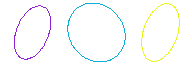Color ellipses used for input

I thought these shapes would be a trivial task for the GAN to generate, but I was of course mistaken.

After implementing the DCGAN network based on the DCGAN tutorial, my first attempt that actually did something produced color in some kind of shape but not actual ellipses.

A note on the images shown throughout this post: Let’s say we have 10 thousand images in our dataset (in this case 10 thousand images of an ellipse). One epoch consists of running through all these images once and a network is trained for 50 epochs. At the end of each epoch, an image is captured based on 16 sample inputs to the generator. These inputs stay the same during training. Thus, we have 50 images (one for each epoch) with 16 generated samples when the network is done training, and we are ideally interested in seeing these 16 images get more realistic over time.

The video below shows the evolution of one of these network training sessions. The video is stitched together from the 50 epoch images. Notice that at the beginning of training, the output of the generator is a gray blob which is the random data. Over time, some colors emerge, until training collapses in the end and it just generates white backgrounds :-)

### Ellipses in opaque black and white

Taking a step back and reviewing the tutorial again, I took note of a few things that I did not pay attention to initially:

1. The tutorial uses white, opaque digits on a black background. I was using unfilled (not opaque) ellipses on a white background.
2. The images are only black and white (grayscale). I was using many colors.
3. The MNIST dataset consists of 60 thousand examples. I was using a few hundred images.

If the goal of the generator is to fool the discriminator, but the images of ellipses are actually mostly white background with a little bit of color, it makes somewhat intuitive sense that the generator ends up just drawing white backgrounds as seen in the video above.

With this in mind, I created 10 thousand opaque white ellipses on a black background, just to prove that the network was indeed working. Here are some examples:Opaque ellipses, black and white

The result from doing this was much better, and the generator ended up creating something that resembles circles:

Wow, I created a neural network with 1 million parameters that can generate white blobs on a black background *crowd goes wild and gives a standing ovation*.

Sarcasm aside, it is always a good feeling when the network finally does something within a reasonable timeframe (it took about a minute to train this network).

### Deeper, wider, opaque, color

After the “success” of the black and white ellipses, I started reviewing some tips on how to tweak a GAN (see references at the bottom of post). Without going into too much detail, I basically made the neural network slightly deeper (more layers) and slightly wider (more features) and switched back to using random colors for the ellipses, while keeping them opaque.

Here are some examples of the input ellipses:Opaque ellipses, with color

After training the network with these images, it was interesting to see the 16 generated samples converge to colored blobs and then change dramatically between epochs. I think this is what is known as “mode collapse” and is a known issue/risk when training GANs:

Each iteration of [the] generator over-optimizes for a particular discriminator, and the discriminator never manages to learn its way out of the trap. As a result the generators rotate through a small set of output types. This form of GAN failure is called mode collapse.

Google Developers, Common Problems with GANs

Mode collapse is most obvious when viewing the epoch images individually, so rather than stitch them together into a video, I have included 50 images below. Notice that after about 20-25 epochs, the output starts to resemble colored ellipses, and all epochs after that do not seem to improve much:

I must admit, I think there’s a certain beauty to these generated images, but to be honest, it is still just randomly colored blobs, and they could be generated with much simpler algorithms than this beast of a neural network.

### Generating cartoon avatars

Instead of continuing to tweak the ellipses-generating network, I wanted to see if I could generate more complex images. My original idea was to generate cartoon like images, and to my great delight, Google provides the Cartoon Set, a dataset consisting of thousands of cartoon avatars, licensed under the CC-BY license.

You have already seen an example result of using this dataset at the top of this post. Here are the 50 epoch images from training the network on the small version of the dataset (10 thousand images). Notice that the network starts to create face-like images after just a few epochs, and then starts cycling the style of the face, probably due to the above mentioned mode collapse.:

This is as far as I got currently. I would like to create a little web app for generating these images in the browser, but that will have to wait for another day. It would also be nice to be able to provide the facial features (hair color, eye color, etc.) as inputs to the network and see how that performs.

To keep my motivation up though, I think I need to switch gears and try something else for now. This was fun! :-)

### References

A search for “DCGAN Tensorflow” yields many useful results, a lot of which I have skimmed as well, but the above are the primary resources.

Categories

## Reinforcement learning

I have been looking into a machine learning technique called reinforcement learning (RL) lately. This was on my TODO for a while, and I must say, this field is incredibly exciting! I played around with some OpenAI Gym environments and re-implemented two RL algorithms mostly based on code I found from other authors.

After spending many hours on this, I can still only get my algorithm to solve the Cartpole problem, where the goal is to balance a pole on a moving cart (video below). I haven’t cracked the nut on a continuous action problem like Pendulum, where the goal is to swing the pendulum into an upright position and keep it there (video below).

Anyway, here is my implementation of the RL algorithms. Perhaps it will be useful for someone :-)